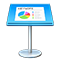# Add an equation with MathType

If you have MathType 6.7d or later installed, you can use it to include mathematical expressions and equations in a presentation.

## Use MathType to add mathematical expressions and equations

1. Click where you want the equation to appear.

2. Choose Insert > MathType Equation (from the Insert menu at the top of your screen).

3. Type or paste your equation in the field provided.

See MathType Help for instructions on using the MathType tools.

4. To save your equation, choose File > “Close and Return to Keynote” (from the MathType File menu at the top of your screen), then click Yes in the window that appears.

The equation appears at the insertion point in your presentation. To edit the equation, double-click it to open MathType again.

You can change the appearance of the equation just as you can other images in your presentation.